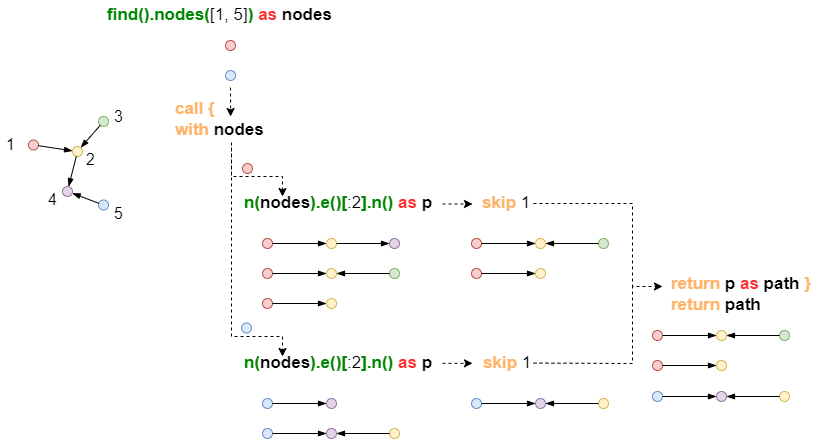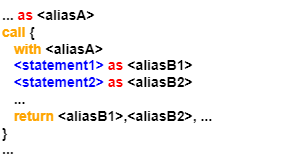# Change Nickname

Current Nickname:
Search
v4.0
v4.0

# CALL | Subquery (V4.1)

V4.1

## Format, Parameters

CALL executes subquery in a UQL statment. This subquery starts with WITH and ends with RETURN, and it applys query and calculation to each row of the input data stream separately, other than query all the input rows and then calculate all the results combinedly. Subquery helps caluses such as GROUP BY, ORDER BY, LIMIT, SKIP, and aggretation funtions and `distinct()` when processing the results of a single execution of a query statement in the UQL.

Syntax:

• Format:
call {
with `<columnA1>`, `<columnA2>`, ...
...
return `<columnB1>`, `<columnB2>`, ...
}
• Parameters: see table below
• Affected columns: `<columnA>` and all its homologous columns; when aliases are non-homologous columns, they will be trimmed to the shortest lengths to become homologous columns before calculation
Name Category Specification Description
`<columnA>` NODE，EDGE，PATH，ATTR，ARRAY，TABLE / The returned data columns from the major UQL query statement
`<columnB>` NODE，EDGE，PATH，ATTR，ARRAY，TABLE / The returned data columns from a subsequent UQL query statement, the alias must not be the same as the major one; Can be used by major UQL query statements following

## Analysis

``````find().nodes([1, 5]) as nodes
call {
with nodes
n(nodes).e()[:2].n() as p
limit 2
return p as path
}
return path
``````

In the UQL statement above, CALL splices data columns from a major query statement into multiple sub queries and sets row number limits to each sub query's result respectively## Single Query in Subquery

Example: do not use GROUP BY, find how many cards Customer CU001, CU002, and CU003 hold respectively``````uncollect ["CU001", "CU002", "CU003"] as user
call {
with user
n({_id == user}).e({@has}).n({@card} as n)
return count(n) as number
}
return user, number
``````

Analysis: CALL aggregates each `user`'s `n` to get `number`, i.e. group all `user`s `n` based on `user` and aggregate all `user`s `n` in group.

## Multiple Queries in Subquery

Example: find 10 transfer edges with an amount higher than 8,000 and rate each edge: if there is no transfer between its innitial node and terminal node within 2 steps, then add 0.5 points to the two nodes

``````n({@card} as n1).re({@transfer.amount > 8000} as e).n({@card} as n2)
call {
with n1, n2
optional n(n1 as a1).e({@transfer})[:2].n({_id == "CA029"}).limit(1)
with CASE a1 when 0 then 0 else 1 END as c1
optional n(n2 as a2).e({@transfer})[:2].n({_id == "CA029"}).limit(1)
with CASE a2 when 0 then 0 else 1 END as c2
return (c1+c2)*0.5 as c
}
return e{*}, c
``````

Analysis: CALL calculates `c` for each row's `n1` and `n2`; the lengths of `c` is the same as the length of `n1` and `n2`'s homologous column `b`.